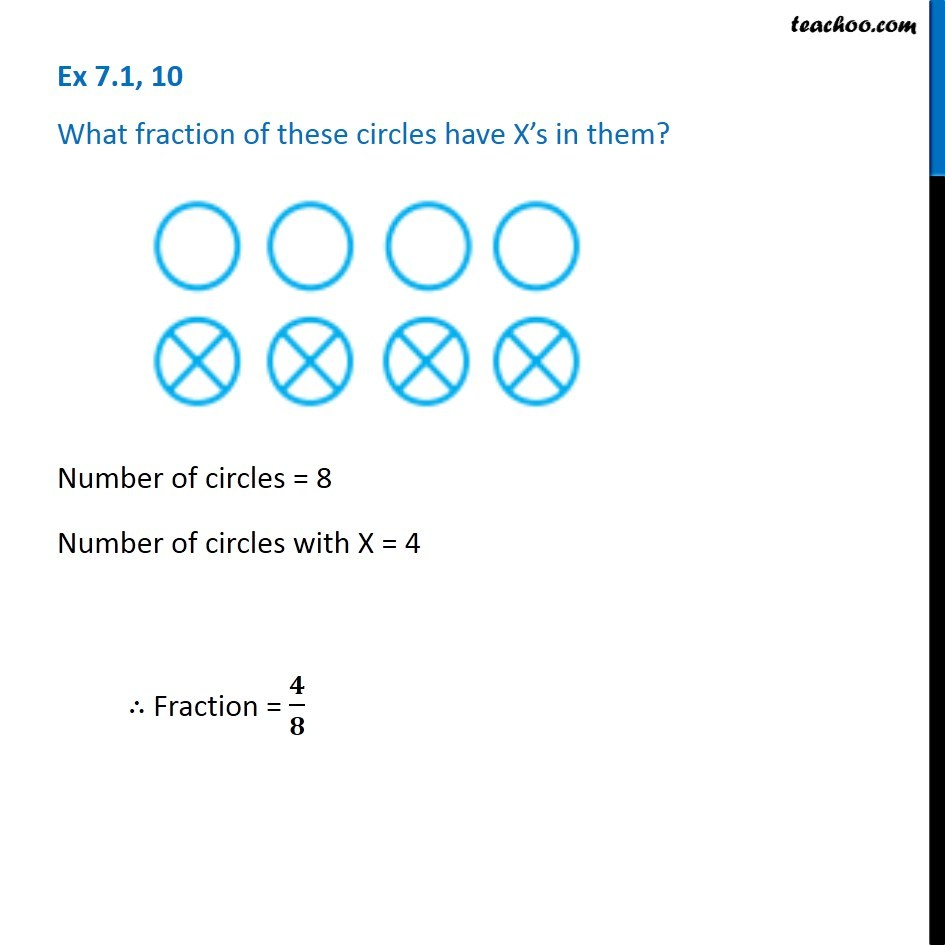1. Chapter 7 Class 6 Fractions
2. Concept wise
3. Finding fraction

Transcript

Ex 7.1, 10 - Chapter 7 Class 6 Fractions - NCERT Book What fraction of these circles have X’s in them? Number of circles = 8 Number of circles with X = 4 ∴ Fraction = 4/8

Finding fraction